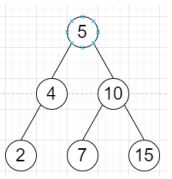# Program to convert level order binary tree traversal to linked list in Python

Suppose we have a binary search tree, we have to convert it to a singly linked list using levelorder traversal.

So, if the input is likethen the output will be [5, 4, 10, 2, 7, 15, ]

To solve this, we will follow these steps −

• q := a list with value root

• while q is not empty, do

• curr := delete first element from q

• if curr is not null, then

• next of currNode := a new linked list node with value of curr

• currNode := next of currNode

• insert left of curr at the end of q

• insert right curr at the end of q

Let us see the following implementation to get better understanding −

## Example

class ListNode:
def __init__(self, data, next = None):
self.val = data
self.next = next
class TreeNode:
def __init__(self, data, left = None, right = None):
self.val = data
self.left = left
self.right = right

print('[', end = "")
while ptr:
print(ptr.val, end = ", ")
ptr = ptr.next
print(']')

class Solution:
def solve(self, root):
q = [root]
while q:
curr = q.pop(0)
if curr:
currNode.next = ListNode(curr.val)
currNode = currNode.next
q.append(curr.left)
q.append(curr.right)

ob = Solution()
root = TreeNode(5)
root.left = TreeNode(4)
root.right = TreeNode(10)
root.left.left = TreeNode(2)
root.right.left = TreeNode(7)
root.right.right = TreeNode(15)
print_list(head)

## Input

root = TreeNode(5)
root.left = TreeNode(4)
root.right = TreeNode(10)
root.left.left = TreeNode(2)
root.right.left = TreeNode(7)
root.right.right = TreeNode(15)
head = ob.solve(root)

## Output

[5, 4, 10, 2, 7, 15, ]